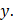# There are two numbers such that the sum of twice the first and thrice the second is 39, while the sum of thrice the first and twice the second is 36. The larger of the two is    (a) 6 (b) 8 (c) 9 (d) 12

## Question ID - 54090 :- There are two numbers such that the sum of twice the first and thrice the second is 39, while the sum of thrice the first and twice the second is 36. The larger of the two is    (a) 6 (b) 8 (c) 9 (d) 12

3537

Let the numbers beandThen,On solvingwe getLargest number = 9.

Next Question :
 The ratio of the radii of gyration of a circular disc about a tangential axis in the plane of the disc and of a circular ring of the same radius about a tangential axis in the plane of the ring is a)b)c)d)